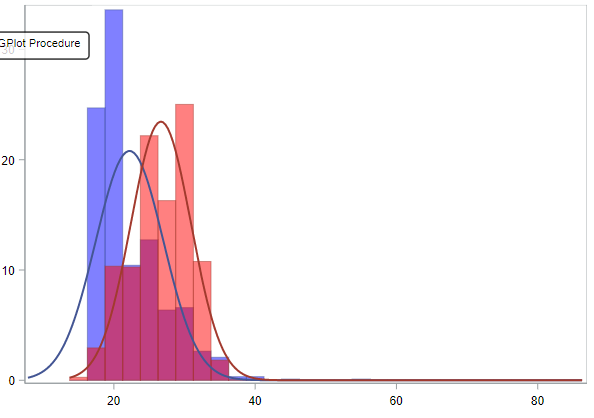## Using slider to set cutoff for grouped histogram

I am trying to create a histogram to show how the age distributions differ between customers in two groups, e.g. high and low probability of buying a product. Ideally, I want to use a slider to dynamically change the score cutoff, so that the user can see how the two distributions look for e.g. scores above\below 0.9, 0.5, etc. I have a more static HTML based report that illustrates the two different distribution, although for a fixed cutoff. Is there a way to create two groups based on a slider or other user input?1 ACCEPTED SOLUTION

Accepted SolutionsSam_SAS
SAS Super FREQ

## Re: Using slider to set cutoff for grouped histogram

Hello,

Did you try creating a slider that filters the histogram? If you assign your score variable to the slider, it seems like this should give you the desired result.

Or did you want your group variable to be defined by the slider setting? This could be accomplished by assigning a parameter to the slider and then creating a calculated measure based on that parameter.

Let us know if that helps, or if I am misunderstanding what you want to do. If you could show a row or two of your data, that would help us understand.

Thanks,

Sam

5 REPLIES 5Sam_SAS
SAS Super FREQ

## Re: Using slider to set cutoff for grouped histogram

Hello,

Did you try creating a slider that filters the histogram? If you assign your score variable to the slider, it seems like this should give you the desired result.

Or did you want your group variable to be defined by the slider setting? This could be accomplished by assigning a parameter to the slider and then creating a calculated measure based on that parameter.

Let us know if that helps, or if I am misunderstanding what you want to do. If you could show a row or two of your data, that would help us understand.

Thanks,

Sam

## Re: Using slider to set cutoff for grouped histogram

Yes, I want my group variable to be based on the slider setting.

Will try that out, thanks.

## Re: Using slider to set cutoff for grouped histogram

What would the calculated measure look like?

I assume something like if parameter < slider value then group 1 else group 2, but I can't quite see how to write in in VA

## Re: Using slider to set cutoff for grouped histogram

Figured it out:

The calculated item had to be

IF ( 'Scorevariable'n >= 'cutoff parameter'p )
RETURN 1
ELSE 0Sam_SAS
SAS Super FREQ

## Re: Using slider to set cutoff for grouped histogram

Great! Thanks for following up with us. 🙂

The calculated expression syntax in VA is a bit unusual, but it can be very useful.

Sam

Discussion stats
• 5 replies
• 506 views
• 0 likes
• 2 in conversation